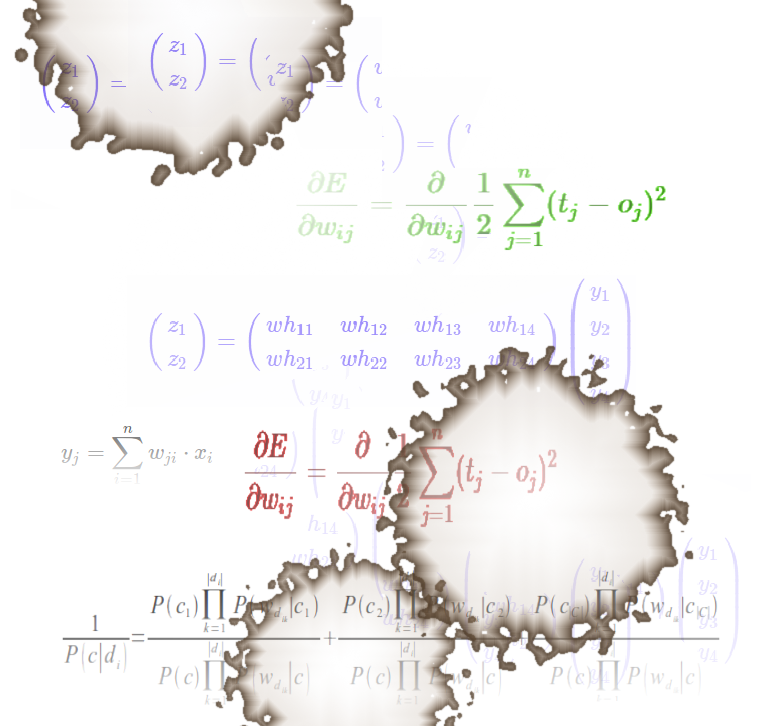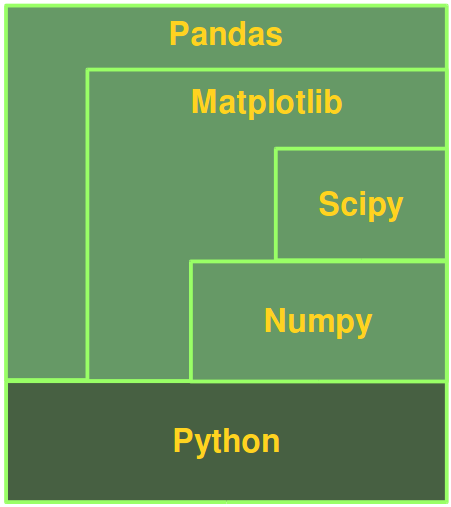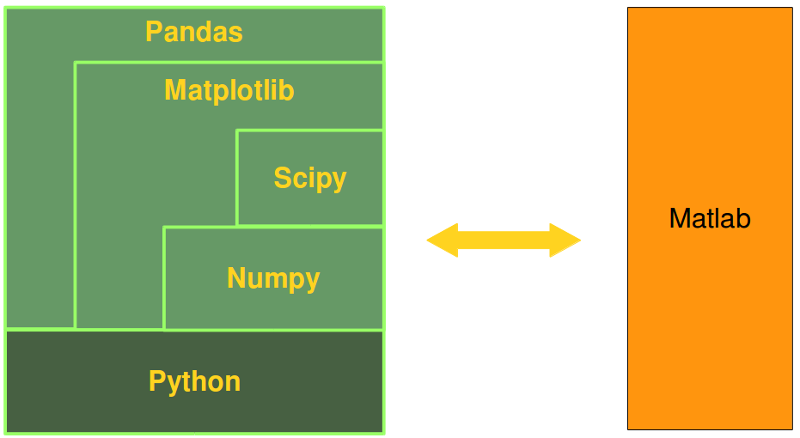## Numerical Programming with Python

### Numerical Programming Definition

The term "Numerical Computing" - a.k.a. numerical computing or scientific computing - can be misleading. One can think about it as "having to do with numbers" as opposed to algorithms dealing with texts for example. If you think of Google and the way it provides links to websites for your search inquiries, you may think about the underlying algorithm as a text based one. Yet, the core of the Google search engine is numerical. To perform the PageRank algorithm Google executes the world's largest matrix computation.

Numerical Computing defines an area of computer science and mathematics dealing with algorithms for numerical approximations of problems from mathematical or numerical analysis, in other words: Algorithms solving problems involving continuous variables. Numerical analysis is used to solve science and engineering problems.

### Data Science and Data Analysis

This tutorial can be used as an online course on Numerical Python as it is needed by Data Scientists and Data Analysts.

Data science is an interdisciplinary subject which includes for example statistics and computer science, especially programming and problem solving skills. Data Science includes everything which is necessary to create and prepare data, to manipulate, filter and clense data and to analyse data. Data can be both structured and unstructured. We could also say Data Science includes all the techniques needed to extract and gain information and insight from data.

Data Science is an umpbrella term which incorporates data analysis, statistics, machine learning and other related scientific fields in order to understand and analyze data.

Another term occuring quite often in this context is "Big Data". Big Data is for sure one of the most often used buzzwords in the software-related marketing world. Marketing managers have found out that using this term can boost the sales of their products, regardless of the fact if they are really dealing with big data or not. The term is often used in fuzzy ways.

Big data is data which is too large and complex, so that it is hard for data-processing application software to deal with them. The problems include capturing and collecting data, data storage, search the data, visualization of the data, querying, and so on.

The following concepts are associated with big data:

• volume:
the sheer amount of data, whether it will be giga-, tera-, peta- or exabytes
• velocity:
the speed of arrival and processing of data
• veracity:
uncertainty or imprecision of data
• variety:
the many sources and types of data both structured and unstructured

The big question is how useful Python is for these purposes. If we would only use Python without any special modules, this language could only poorly perform on the previously mentioned tasks. We will describe the necessary tools in the following chapter.

### Connections between Python, Numpy, Matplotlib, Scipy and PandasPython is a general-purpose language and as such it can and it is widely used by system administrators for operating system administration, by web developpers as a tool to create dynamic websites and by linguists for natural language processing tasks. Being a truely general-purpose language, Python can of course - without using any special numerical modules - be used to solve numerical problems as well. So far so good, but the crux of the matter is the execution speed. Pure Python without any numerical modules couldn't be used for numerical tasks Matlab, R and other languages are designed for. If it comes to computational problem solving, it is of greatest importance to consider the performance of algorithms, both concerning speed and data usage.

If we use Python in combination with its modules NumPy, SciPy, Matplotlib and Pandas, it belongs to the top numerical programming languages. It is as efficient - if not even more efficient - than Matlab or R.Numpy is a module which provides the basic data structures, implementing multi-dimensional arrays and matrices. Besides that the module supplies the necessary functionalities to create and manipulate these data structures. SciPy is based on top of Numpy, i.e. it uses the data structures provided by NumPy. It extends the capabilities of NumPy with further useful functions for minimization, regression, Fourier-transformation and many others.

Matplotlib is a plotting library for the Python programming language and the numerically oriented modules like NumPy and SciPy.

The youngest child in this family of modules is Pandas. Pandas is using all of the previously mentioned modules. It's build on top of them to provide a module for the Python language, which is also capable of data manipulation and analysis. The special focus of Pandas consists in offering data structures and operations for manipulating numerical tables and time series. The name is derived from the term "panel data". Pandas is well suited for working with tabular data as it is known from spread sheet programming like Excel.

### Python, An Alternative to Matlab

Python is becoming more and more the main programming language for data scientists. Yet, there are still many scientists and engineers in the scientific and engineering world that use R and MATLAB to solve their data analysis and data science problems. It's a question troubling lots of people, which language they should choose: The functionality of R was developed with statisticians in mind, whereas Python is a general-purpose language. Nevertheless, Python is also - in combination with its specialized modules, like Numpy, Scipy, Matplotlib, Pandas and so, - an ideal programming language for solving numerical problems. Furthermore, the community of Python is a lot larger and faster growing than the one from R.

The principal disadvantage of MATLAB against Python are the costs. Python with NumPy, SciPy, Matplotlib and Pandas is completely free, whereas MATLAB can be very expensive. "Free" means both "free" as in "free beer" and "free" as in "freedom"! Even though MATLAB has a huge number of additional toolboxes available, Python has the advantage that it is a more modern and complete programming language. Python is continually becoming more powerful by a rapidly growing number of specialized modules.

Python in combination with Numpy, Scipy, Matplotlib and Pandas can be used as a complete replacement for MATLAB.